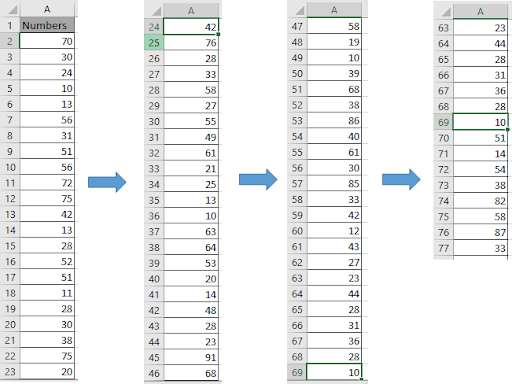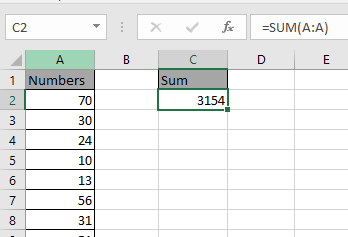# How to Sum across entire column in Excel

In this article, we will learn how to get the sum or add cells across entire column in Excel.

Sometimes we need to access the entire column in Excel 2016.
In this article, we will learn how to sum the values in the entire column in excel 2016. We will use the SUM function to add numbers.

SUM function adds up the values.
SUM = number 1 + number 2 + …

Syntax:

=SUM(number 1, number 2, ..)

Let’s understand how to add cells in excel 2016 with the example explained here.
Here we have 76 numbers in column A.Here we need to sum the values present in this column their would two methods
Use the formula

=SUM(A2:A77)
OR
=SUM(A:A)Sum function add up the numbers in the list and returns the Sum.

Hope you understand how to use SUM function in Excel to calculate sum across entire column. Explore more articles on Excel Mathematical functions here. Please state your queries in the comment box below.

Related Articles:

How to Sum across sheets in Excel

2 Ways to Sum by Month in Excel

Popular Articles:

50 Excel Shortcuts to Increase Your Productivity

How to use the VLOOKUP Function in Excel

How to use the COUNTIF function in Excel

How to use the SUMIF Function in Excel

Terms and Conditions of use

The applications/code on this site are distributed as is and without warranties or liability. In no event shall the owner of the copyrights, or the authors of the applications/code be liable for any loss of profit, any problems or any damage resulting from the use or evaluation of the applications/code.